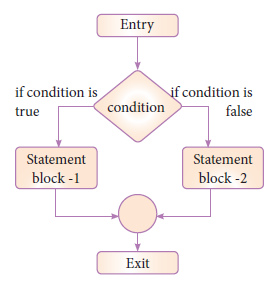#### if...else Statement in Pythpn

The if .. else statement provides control to check the true block as well as the false block. Following is the syntax of ‘if..else’ statement.

Syntax:

`if <condition>:statements-block 1else:statements-block 2`if..else statement thus provides two possibilities and the condition determines which BLOCK is to be executed.

Example : #Program to check if the accepted number odd or even

`a = int(input("Enter any number :"))if a%2==0:print (a, " is an even number")else:print (a, " is an odd number")`

Output 1:

Enter any number :56

56 is an even number

Output 2:

Enter any number :67

67 is an odd number

An alternate method to rewrite the above program is also available in Python. The complete if..else can also written as:

Syntax:

`variable = variable1 if condition else variable 2`

Example : #Program to check if the accepted number is odd or even (using alternate method of if...else)

`a = int (input("Enter any number :"))x="even" if a%2==0 else "odd"print (a, " is ",x)`

Output 1:

Enter any number :3

3 is odd

Output 2:

Enter any number :22

22 is even